Question

# Draw the ray diagram: An object at a distance of 4 cm from a convex lens...

Draw the ray diagram: An object at a distance of 4 cm from a convex lens whose focal length is 2 cm. The the object be 1 cm tall.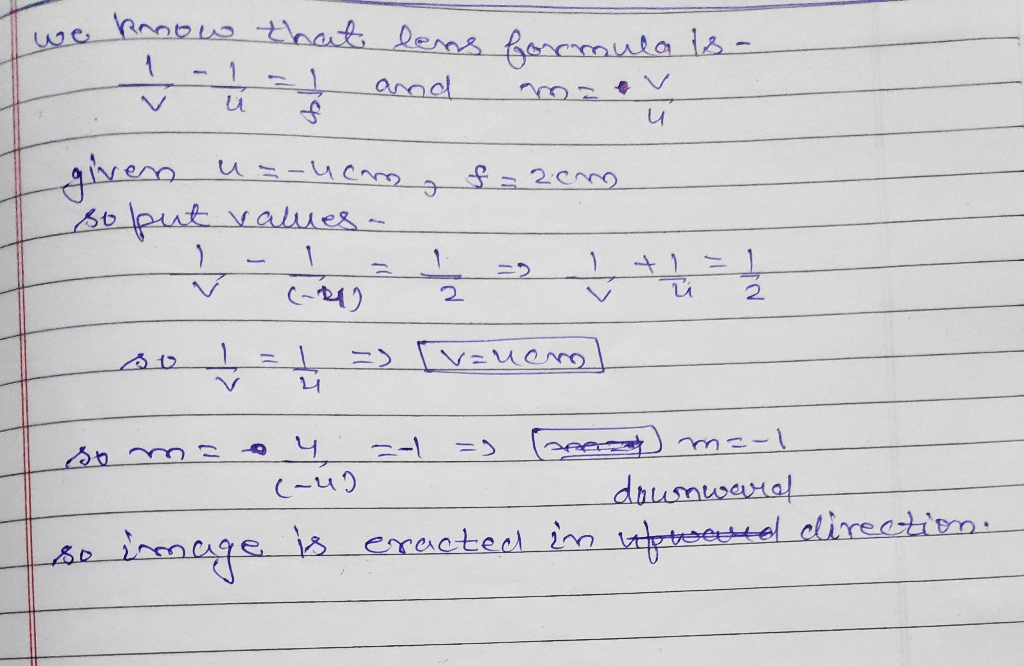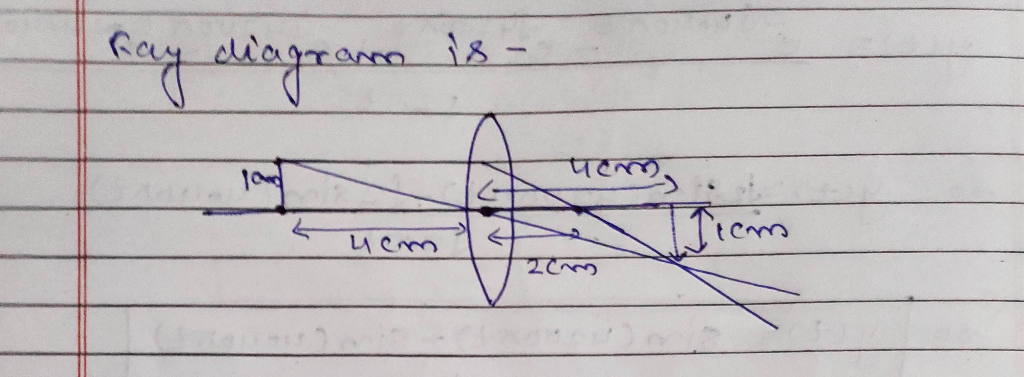#### Earn Coins

Coins can be redeemed for fabulous gifts.

Similar Homework Help Questions
• ### An object is placed 40 cm to the left of a convex lens that has a...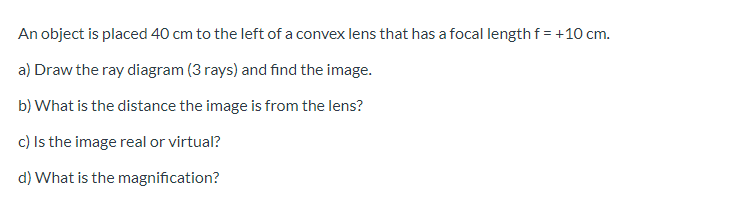An object is placed 40 cm to the left of a convex lens that has a focal length f= +10 cm. a) Draw the ray diagram (3 rays) and find the image. b) What is the distance the image is from the lens? c) Is the image real or virtual? d) What is the magnification?

• ### An object is placed 40 cm to the left of a convex lens that has a...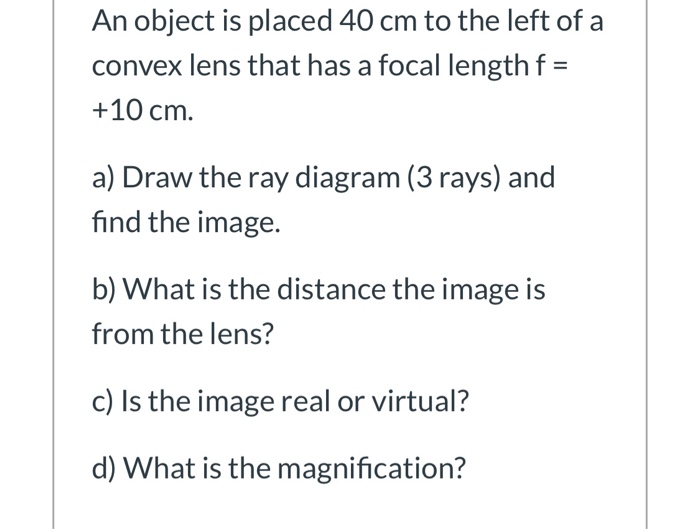An object is placed 40 cm to the left of a convex lens that has a focal length f = +10 cm. a) Draw the ray diagram (3 rays) and find the image. b) What is the distance the image is from the lens? c) Is the image real or virtual? d) What is the magnification?

• ### The focal length of a convex lens is given to be 17 cm. It's magnification is...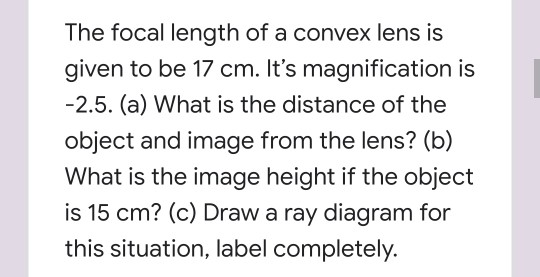The focal length of a convex lens is given to be 17 cm. It's magnification is -2.5. (a) What is the distance of the object and image from the lens? (b) What is the image height if the object is 15 cm? (c) Draw a ray diagram for this situation, label completely.

• ### For the following question, draw a ray diagram for each lens draw a careful and accurate...

For the following question, draw a ray diagram for each lens draw a careful and accurate ray diagram of this image (from lens 1) as an object (for lens 2) forming an image through the second lens. Finally, draw a careful and accurate ray diagram of the entire situation, including both lenses and all images. An object 5 cm high is located 75 cm from a converging lens (X1 = 75 cm) of focal length f1 = 50 cm. A...

• ### 2. An object is placed a distance of 25.0 cm from a converging lens having a...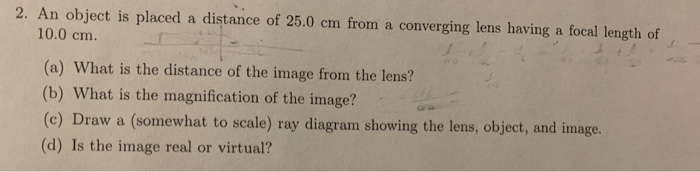2. An object is placed a distance of 25.0 cm from a converging lens having a focal length of 10.0 cm. (a) What is the distance of the image from the lens? (b) What is the magnification of the image? (c) Draw a (somewhat to scale) ray diagram showing the lens, object, and image. (d) Is the image real or virtual?

• ### A 4.50 cm tall object is placed at a distance of (24.8) cm from a convex...

A 4.50 cm tall object is placed at a distance of (24.8) cm from a convex lens with a focal length of (5.25) cm. Find the size of the resulting image. Give your answer in centimeters (cm) with the correct sign and with 3 significant figures.

• ### A 4.00-cm tall object is placed a distance of 48 cm from a concave mirror having a focal length of 16cm.

1. A 4.00-cm tall object is placed a distance of 48 cm from a concave mirror having a focal length of 16cm. Determine the image distance and the image size. 2. A 4.00-cm tall object is placed a distance of 8 cm from a concave mirror having a focal length of 16cm. Determine the image distance and the image size. 3. Determine the image distance and image height for a 5.00-cm tall object. placed 30.0 cm Infront of from a convex mirror...

• ### O . 3) A diverging lens has f= -12 cm. A) Draw a ray diagram and...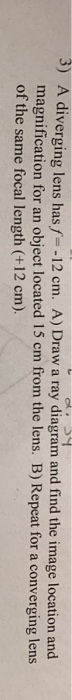O . 3) A diverging lens has f= -12 cm. A) Draw a ray diagram and find the image location and magnification for an object located 15 cm from the lens. B) Repeat for a converging lens of the same focal length (+12 cm).

• ### An object is located 40.0 cm from the front side of a divergent lens. A focal point of the lens is located at 20.0 cm from the front side of the lens. (a) Determine the location of the image form...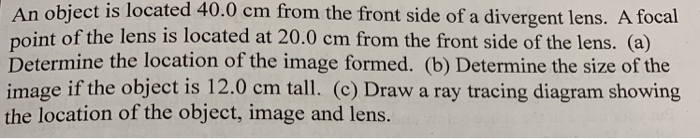An object is located 40.0 cm from the front side of a divergent lens. A focal point of the lens is located at 20.0 cm from the front side of the lens. (a) Determine the location of the image formed. (b) Determine the size of the image if the object is 12.0 cm tall. (c) Draw a ray tracing diagram showing the location of the object, image and lens. An object is located 40.0 cm from the front side of...

• ### Ray Optics 3 Problem Statement A 4.0-cm tall object is placed 50.0 cm from a diverging...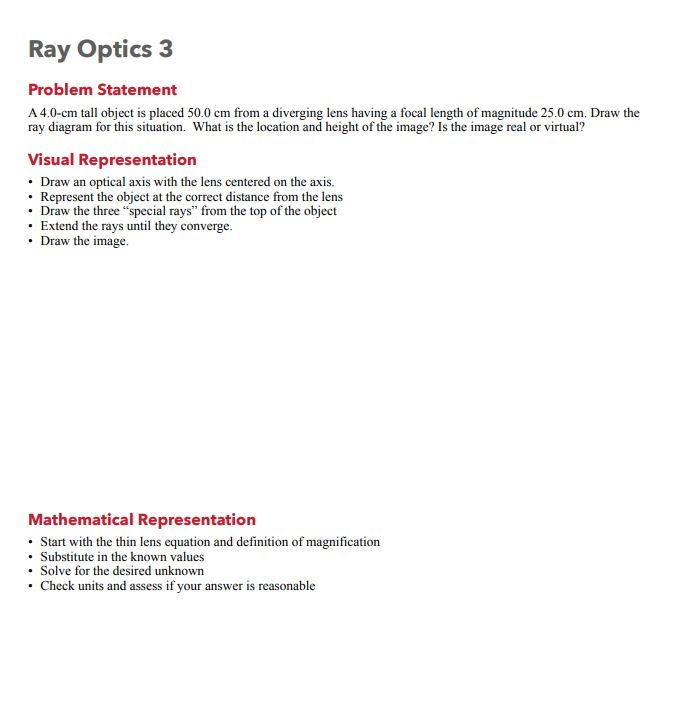Ray Optics 3 Problem Statement A 4.0-cm tall object is placed 50.0 cm from a diverging lens having a focal length of magnitude 25.0 cm. Draw the ray diagram for this situation. What is the location and height of the image? Is the image real or virtual? Visual Representation • Draw an optical axis with the lens centered on the axis. Represent the object at the correct distance from the lens • Draw the three "special rays” from the top...